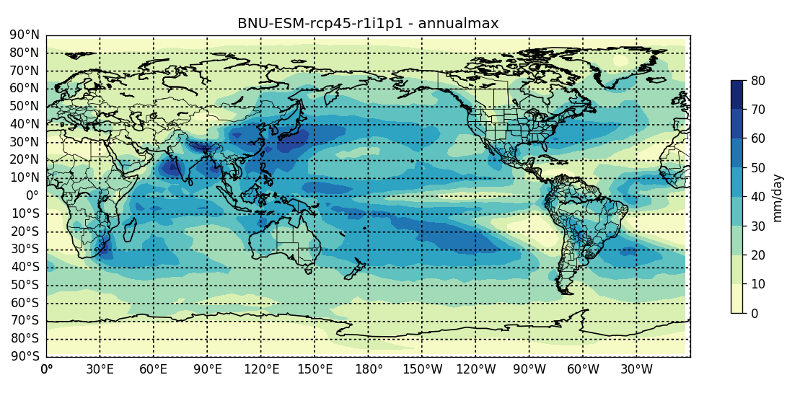# Climate Indices

## Indices

More than 20 climate indices are available in the package, such as the annual number of tropical nights, annual maximum and minimum, etc. You can calculate such indices simply with:

ind = annualmax(C::ClimGrid)

Which returns another ClimGrid. You can also map this ClimGrid with the mapclimgrid function and returns the climatological mean of the annual maximum (e.g. daily precipitation in the example below). A list of indices can be found in the documentation and in the functions.jl source code.

mapclimgrid(ind) # mapping the indice previously calculated## Ensemble mean

You can calculate the ensemble mean with ensemble_mean function, where the input argument is an array of ClimGrids.

Abstract example:

C_model1 = ClimGrid(...) # model #1
C_model2 = ClimGrid(...) # model #2
ens = [C_model1, C_model2] # Create an Array of ClimGrids
E = ensemble_mean(ens) # Returns the mean of all models climatologies

## Climate Indices

Here's a list of climate indices currently provided by ClimateTools. This list may not be always up-to-date. See here for all exported functions.

ClimateTools.annualmaxFunction
annualmax(C::ClimGrid)

Annual maximum of array data.

Let data[i,j] be daily time serie on day i in year j. Extract the highest value for year j.

source
ClimateTools.annualmeanFunction
annualmean(C::ClimGrid)

Annual mean of array data.

Let data[i,j] be daily time serie on day i in year j. Calculate the mean value for year j.

source
ClimateTools.annualminFunction
annualmin(C::ClimGrid)

Annual minimum of array data.

Let data[i,j] be daily time serie on day i in year j. Extract the lowest value for year j.

source
ClimateTools.annualsumFunction
annualsum(C::ClimGrid)

Annual sum of array data.

Let data[i,j] be daily time serie on day i in year j. Sums daily values for year j.

source
ClimateTools.approx_surfacepressureFunction

approxsurfacepressure(sealevelpressure::ClimGrid, orography::ClimGrid, daily_temperature::ClimGrid)

Returns the approximated surface pressure (sp) (Pa) using sea level pressure (psl) (Pa), orography (orog) (m), and daily mean temperature (tas) (K).

$sp = psl * 10^{x}$

where $x = \frac{-orog}{18400 * tas / 273.15}$

source
ClimateTools.customthresoverFunction
customthresover(C::ClimGrid, thres)

customthresover, annual number of days over a specified threshold.

Let TS[i,j] be a daily time serie value on day i in year j. Count the number of days where:

TS[i,j] > thres.

source
ClimateTools.customthresunderFunction
customthresunder(C::ClimGrid, thres)

customthresunder, annual number of days under a specified threshold.

Let TS[i,j] be a daily time serie value on day i in year j. Count the number of days where:

TS[i,j] < thres.

source
ClimateTools.diurnaltemperatureFunction

diurnaltemperature(temperatureminimum::ClimGrid, temperaturemaximum::ClimGrid, α::Float64)

Returns an estimation of the diurnal temperature (temperature between 7:00 (7am) and 17:00 (5pm)). The estimation is a linear combination of the daily minimum temperature (temperatureminimum) and daily maximum temperature (temperaturemaximum). The value of α has to be estimated seperatly from observations and depends on the location. The daily max and min must be in the same unit and in Celsius or Kelvin The diurnal temperature returned is in the same units as the daily minimum temperature and daily maximum temperature.

$Tdiu = α * Tmin + (1 - α) * Tmax$

source
ClimateTools.icingdaysFunction
icingdays(C::ClimGrid)

ID, Number of summer days: Annual count of days when TX (daily maximum temperature) < 0 degree Celsius.

Let TX[i,j] be daily maximum temperature on day i in year j. Count the number of days where:

TX[i,j] < 0 Celsius.

source
ClimateTools.frostdaysFunction
frostdays(C::ClimGrid)

FD, Number of frost days: Annual count of days when TN (daily minimum temperature) < 0 Celsius.

Let TN[i,j] be daily minimum temperature on day i in year j. Count the number of days where:

TN[i,j] < 0 Celsius.

source
ClimateTools.prcp1Function
prcp1(C::ClimGrid)

Annual number with preciptation >= 1 mm. This function returns a ClimGrid. Input data should be in mm.

source
ClimateTools.summerdaysFunction
summerdays(C::ClimGrid)

SD, Number of summer days: Annual count of days when TX (daily maximum temperature) > 25 degree Celsius.

Let TX[i,j] be daily maximum temperature on day i in year j. Count the number of days where:

TX[i,j] > 25 Celsius.

source
ClimateTools.tropicalnightsFunction
tropicalnights(C::ClimGrid)

TropicalNights, Number of tropical nights: Annual count of days when TN (daily maximum temperature) > 20 degree Celsius.

Let TN[i,j] be daily minimum temperature on day i in year j. Count the number of days where:

TN[i,j] > 20 Celsius.

source
ClimateTools.vaporpressureFunction

vaporpressure(surfacepressure::ClimGrid, specifichumidity::ClimGrid)

Returns the vapor pressure (vp) (Pa) based on the surface pressure (sp) (Pa) and the specific humidity (q).

$vp = \frac{q * sp}{q+0.622}$

source

vaporpressure(specifichumidity::ClimGrid, sealevelpressure::ClimGrid, orography::ClimGrid, daily_temperature::ClimGrid)

Returns the vapor pressure (vp) (Pa) estimated with the specific humidity (q), the sea level pressure (psl) (Pa), the orography (orog) (m) and the daily mean temperature (tas) (K). An approximation of the surface pressure is first computed by using the sea level pressure, orography and the daily mean temperature (see approx_surfacepressure). Then, vapor pressure is calculated by:

$vp = \frac{q * sp}{q+0.622}$

source
ClimateTools.wbgtFunction

wbgt(diurnaltemperature::ClimGrid, vaporpressure::ClimGrid)

Returns the simplified wet-bulb global temperature (wbgt) (Celsius) calculated using the vapor pressure (Pa) of the day and the estimated mean diurnal temperature (Celsius; temperature between 7:00 (7am) and 17:00 (5pm)).

$wbgt = 0.567 * Tday + 0.00393 * vp + 3.94$

source